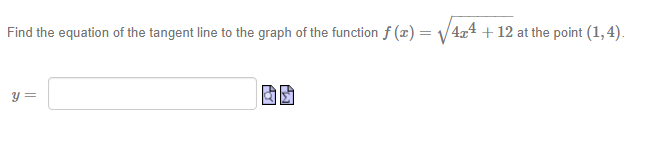# Find the equation of the tangent line to the graph of the function f (x) = v 4,4 + 12 at the point (1,4).

Questionhelp_outlineImage TranscriptioncloseFind the equation of the tangent line to the graph of the function f (x) = v 4,4 + 12 at the point (1,4). fullscreen